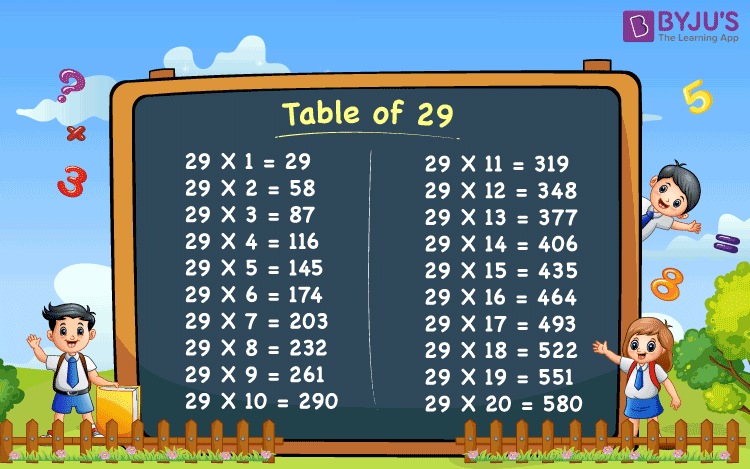# Table of 29

Tables are the basics of Maths we have learned in schools. Table of 29 gives the repeated addition of number 29, repeated for a certain number of times. For example, 5 buckets of 29 bottles each, gives the sum of 29 + 29 + 29 + 29 + 29 = 145. Therefore, the total number of bottles in all the buckets are 145. Or we can also write it as, 5 x 29 = 145, where 5 is the number of buckets and 29 is the number of bottles in each bucket.

## Table of 29 ChartThe multiplication tables are of very useful from Classes 1st to 12th. The calculations we do are sometimes time-consuming as it consists of multiplication problems. Therefore, if we memorize this maths table, we can solve the related problems easily and quickly. Also, aspirants of competitive exams that include maths aptitude questions can memorize 29 for quick calculations.

The table of 29 is given below is little difficult but could be memorised.

## 29 Times Table

Below is the table of 29 from numbers 1 to 20 times.

 29 x 1 = 29 29 x 2 = 58 29 x 3 = 87 29 x 4 = 116 29 x 5 = 145 29 x 6 = 174 29 x 7 = 203 29 x 8 = 232 29 x 9 = 261 29 x 10 = 290 29 x 11 = 319 29 x 12 = 348 29 x 13 = 377 29 x 14 = 406 29 x 15 = 435 29 x 16 = 464 29 x 17 = 493 29 x 18 = 522 29 x 19 = 551 29 x 20 = 580

Download BYJU’S – The learning app and get new ideas of learning Maths and to solve its problems with the help of visuals and interactive videos.

 Get More Maths Table Table of 2 Table of 3 Table of 4 Table of 5 Table of 6 Table of 7 Table of 8 Table of 9 Table of 10 Table of 11 Table of 12 Table of 13 Table of 14 Table of 15 Table of 16 Table of 17 Table of 19 Table of 20
Test your Knowledge on Table of 29